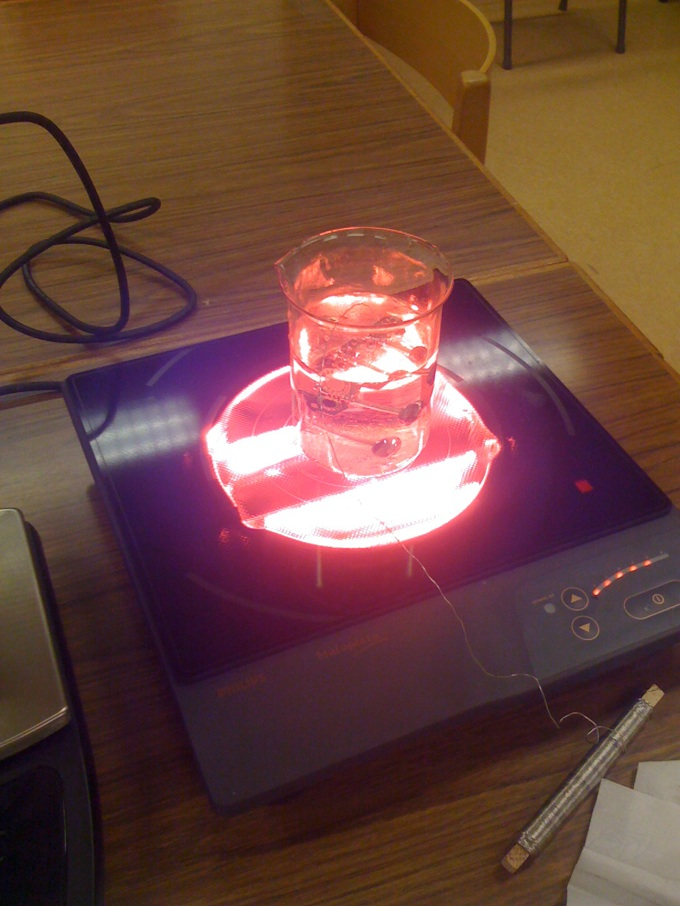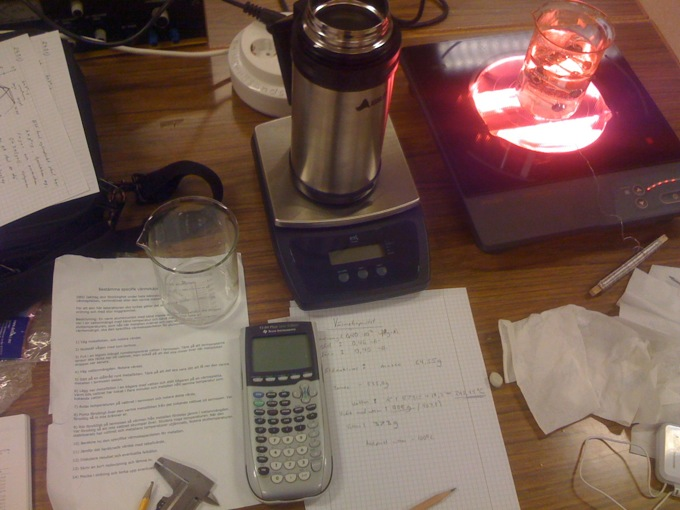# Materials

### To be sure about what materials that the bridge and the tuning pegs are made in, I completed a few experiments.

I started of by checking their density, to see what different kind of metals it could be. I did not have the necessary equipment for calculating their exact volume, but at least I did get some idea.

These are the result from the experiments:

Density:

Tuning pegs
mass: 64,55 g
volume: 2,6mm * pi * (64,4 / 2)^2
= 8469mm^3

p = m / V = (64,55 * 10^-3) / (8469 * 10^-9) = 7621,91522 kg/m^3

Bridge
mass: 51,41 g
volume: 2mm * pi * (32,2)^2
= 6514,657854 mm^3

p = m / V = (51,41 * 10^-3) / (6514 * 10^-9) = 7891,435153 kg/m^3

p - density - brass = 8,4 * 10^-3 kg/m3

Since my calculations are not so accurate it shows that there is a possibility that it is true what the sellers said, that it would be brass.

Here are two pictures from the experiment:Heat capacity:

General:
Weight of the thermos: 535,0 g

Thermodynamics: E = c * m * ΔT
c H2O = 4,19 * 10^3 J / (kg * K)

E (metal) = E (H2O)

Tuning pegs:
Mass: 64,55 g

c * 64,55 * 10^-3 * (78,2) = (4,190 * 10^3) * (372 * 10^-3) * (1,1)
c = 339,66 J / (kg * K)
( ΔT water = 1,1°C )

90 - 535 = 372 g... (weight thermos + water)
100 - 21,8 = 78,2°C

* I believe that this value got so low cause I were to slow when moving the pegs to the thermos...

Bridge:
Mass: 51,4 g

c * (51,4 * 10^-3) * (100 - 22,8) = (4,190 * 10^3) * (371,5 * 10^-3) * (1)
c = 392,2766176 J / (kg * K)
( ΔT water = 1°C )

906,5 - 535 = 371,5 g... (weight thermos + water)

Zink: 0.39 * 10^3 J / (kg * K)
Brass: 0,40 * 10^3 J / (kg * K)
Nickel: 0,44 * 10^3 J / (kg * K)
Iron: 0,45 * 10^3 J / (kg * K)
Steel: 0,46 * 10^3 J / (kg * K)

These are the possible metals that have about the same heat capacity, the only way to make sure would be to do further experiments. Nickel and zink can be excluded since they does not fit with the density. You could perhaps also exclude steel and iron, due to the thermo value. (But we should not forget that there is a probability that it is an alloy.)

Variables and why I did not get a better result.
There may be many reasons to why the results are not better. Other than that the methods may not be the best, the materials may not be made out of the same metal, as for example the saddles on the bridge is probably made out of some other material - the same thing counts for the pegs at the tuners.

In conclusion I can not know for certain what materials they are made out of, without doing more test. One thing that one might do is to test their melting point for example, but that would destroy the parts.